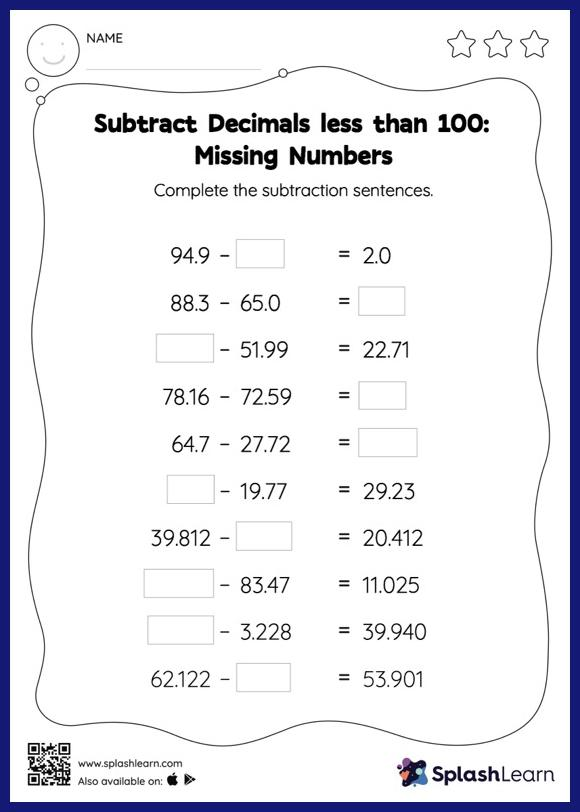# Subtract Decimals less than 100: Missing Numbers Worksheet

Home > Subtract Decimals less than 100: Missing NumbersIn this worksheet, students subtract decimals by aligning the decimal points and using zero as a placeholder. They then use the relationship between addition and subtraction to find the missing number in the subtract decimals less than 100 worksheet. This worksheet is about practicing with the horizontal format in which numbers are written side by side. To develop flexibility with numbers and operations, students need to have enough practice in this format and not just rely on the vertical/column method.